# 南投埔里 楓樺台一渡假村 Tai-Yi Red Maple Resort(Tai-Yi Ecological Leisure Farm)

5,280

• 埔里
• 埔里酒廠

## 選擇專案房型及間數

#### 更改 南投埔里 楓樺台一渡假村 的空房情形及價格

•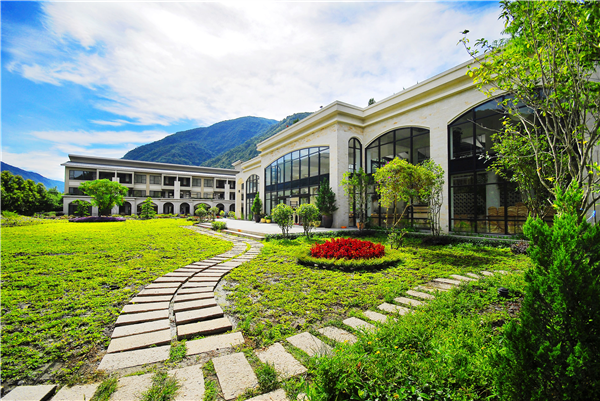酒店外觀
•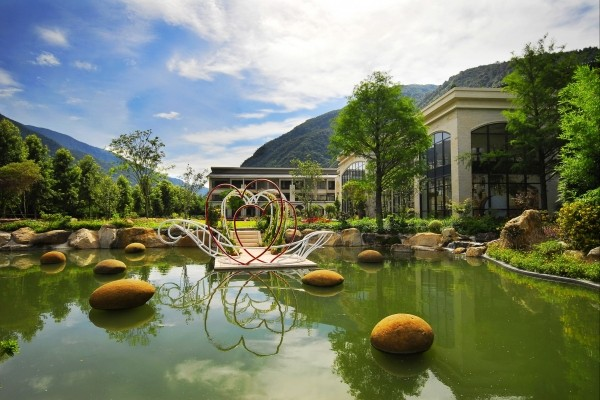環境
•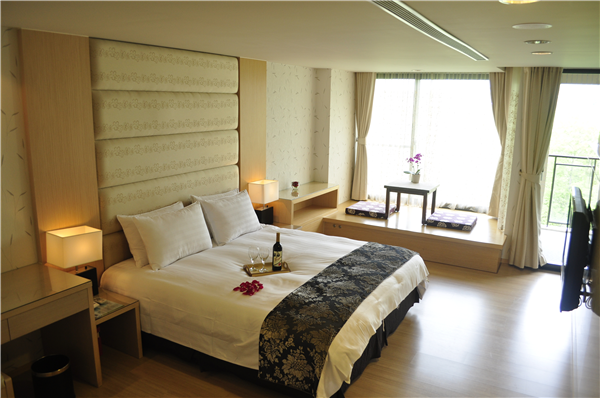客房
•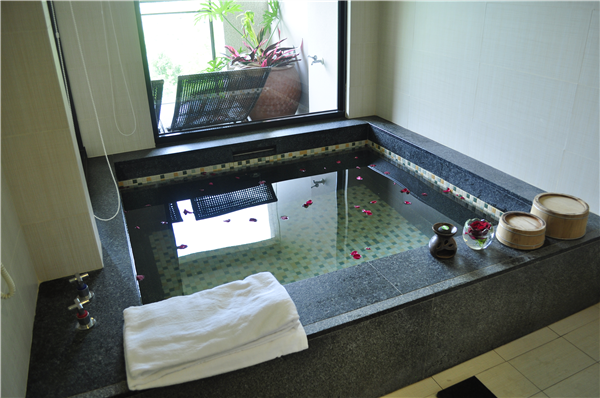衛浴間
•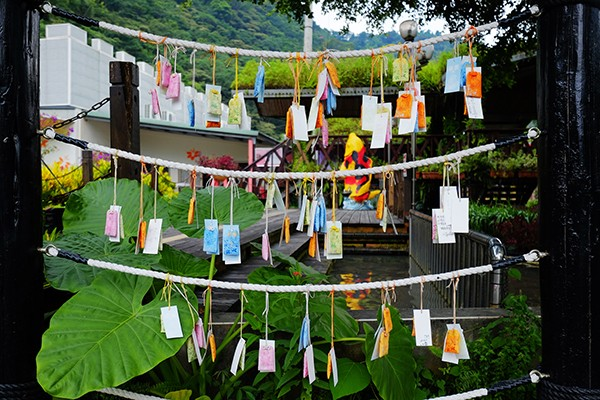環境
•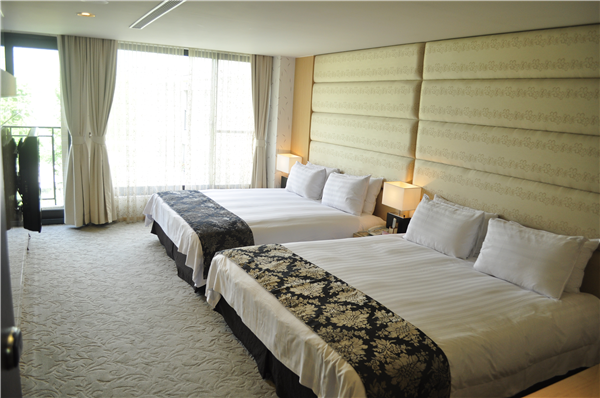客房
•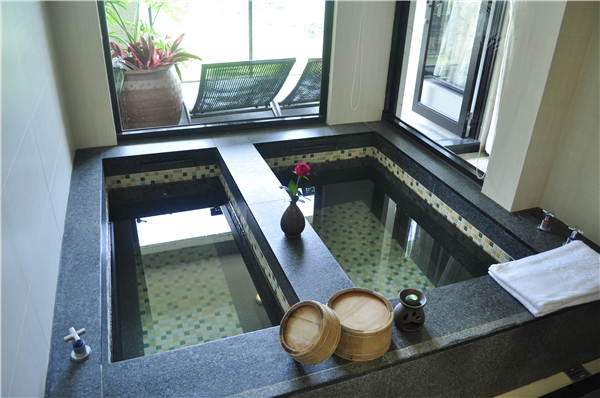衛浴間
•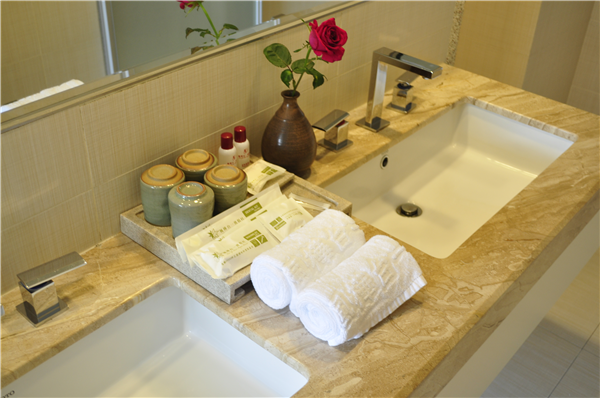衛浴間
•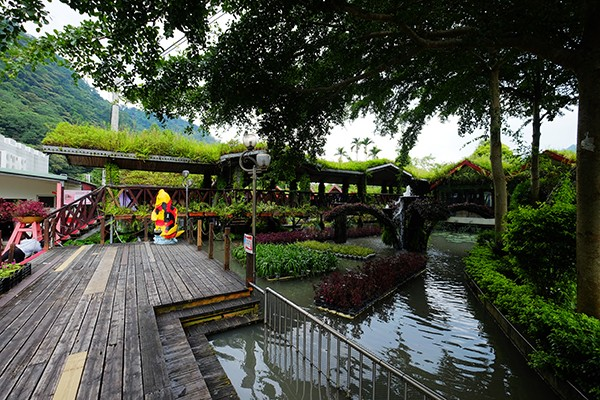環境
•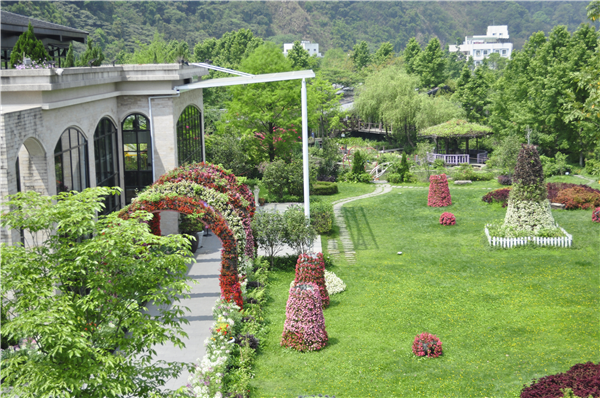花園
•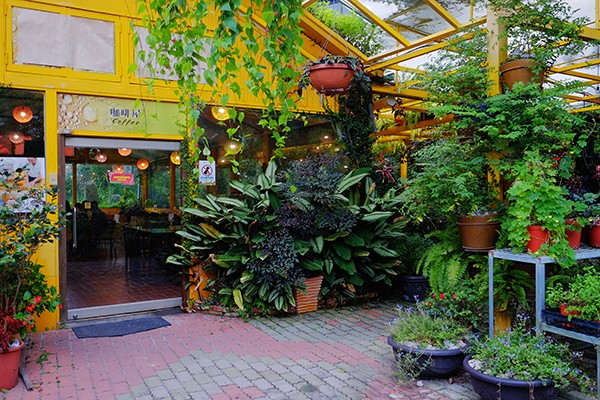環境
•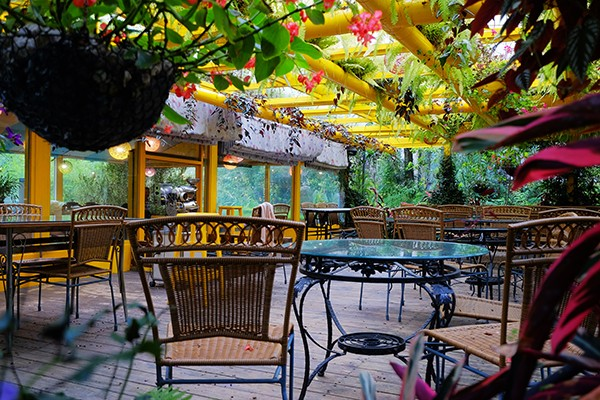環境
•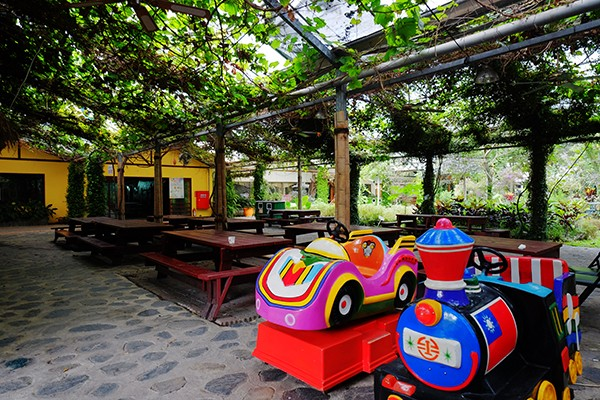環境
•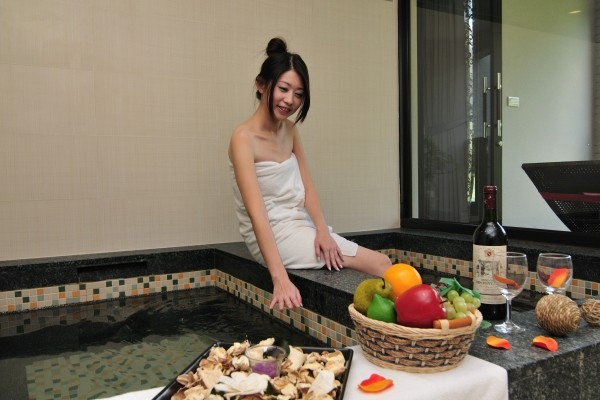環境
•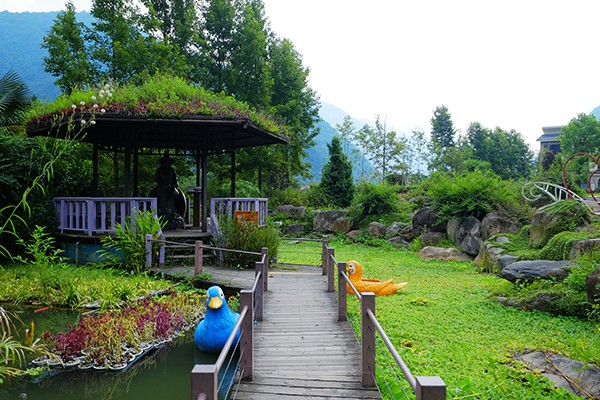環境
•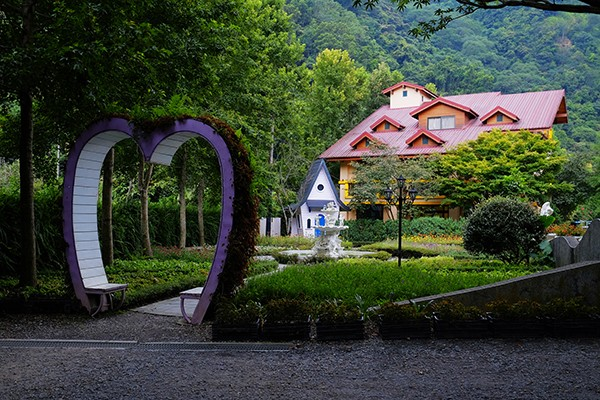環境
•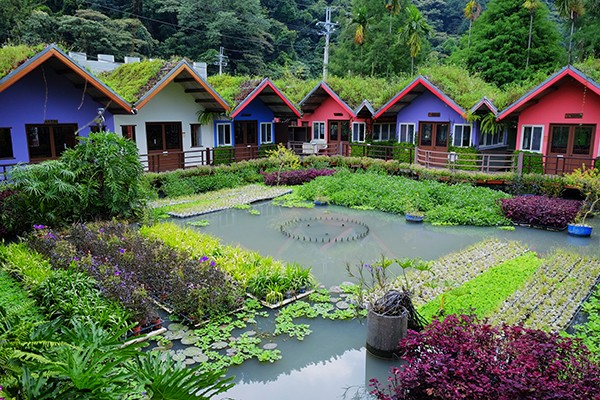環境
•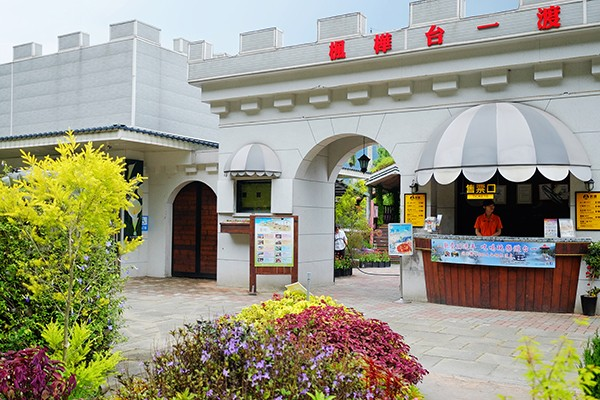環境
•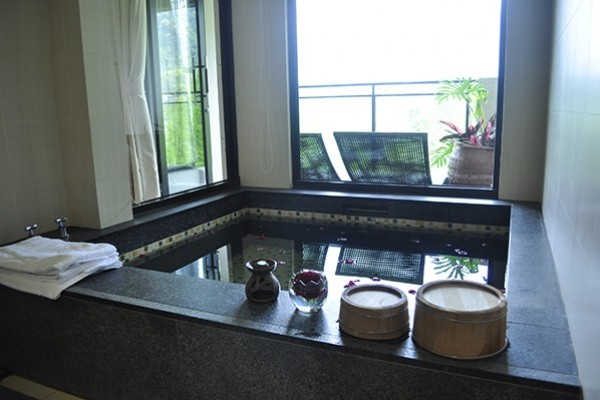環境
•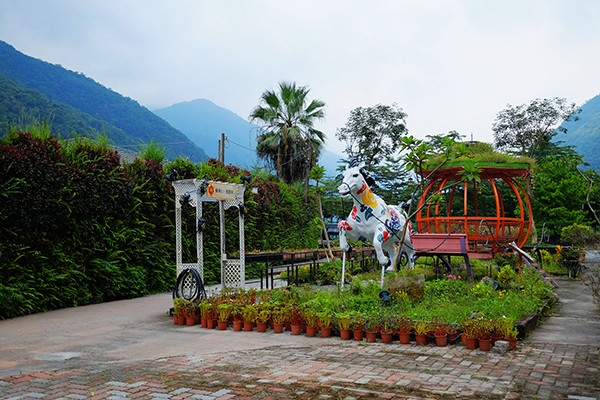環境
•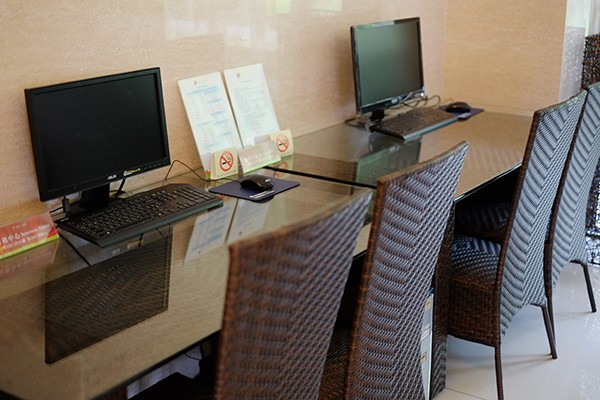環境
•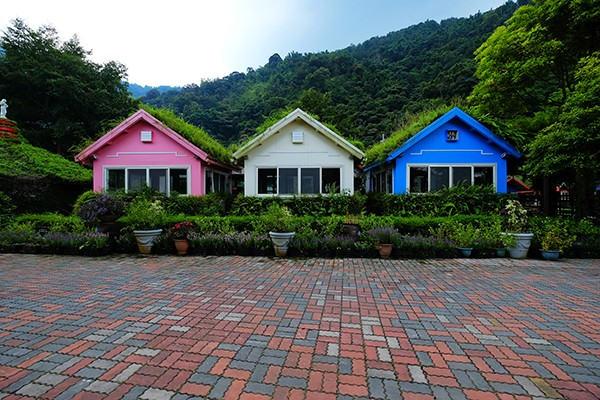環境
•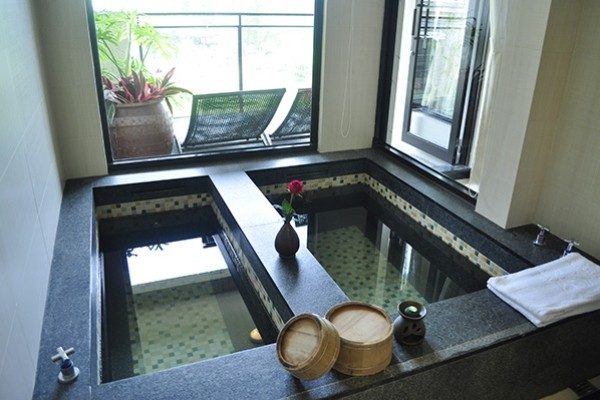環境
•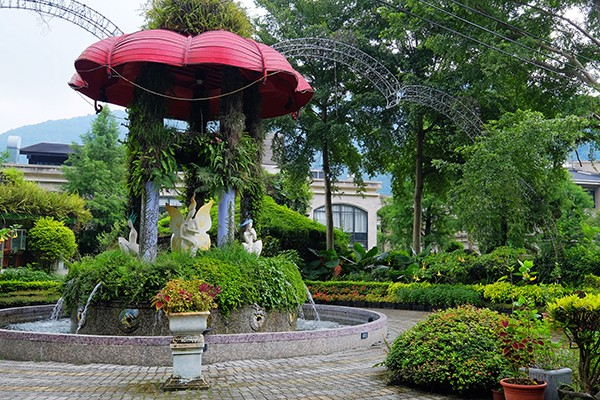環境
•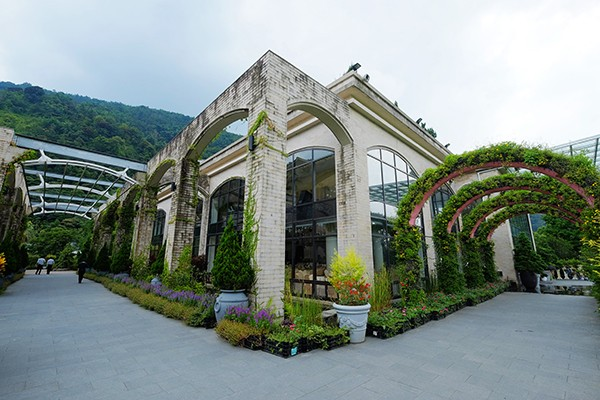環境
•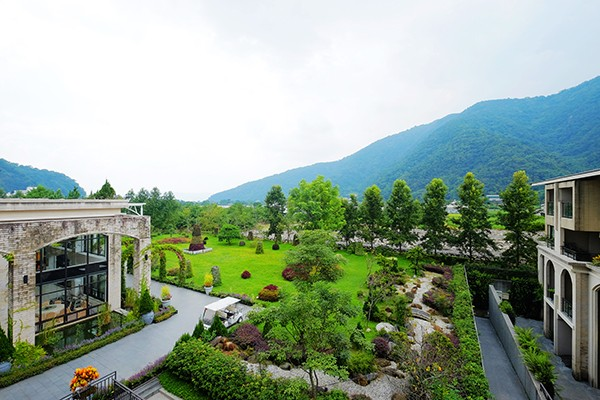環境
•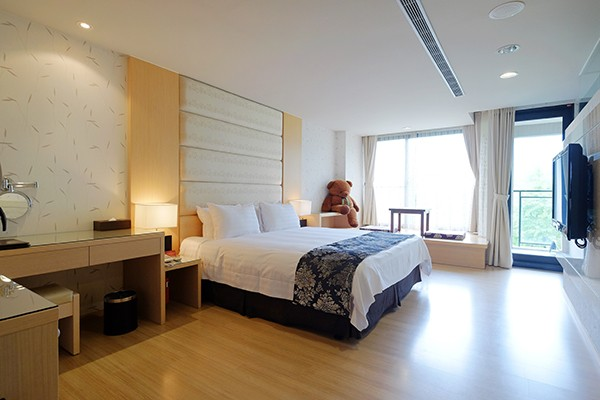環境
•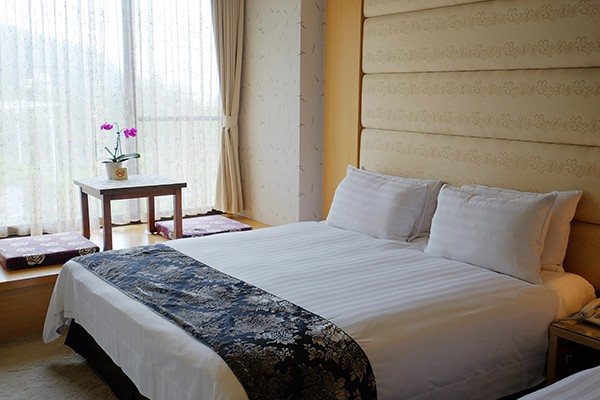環境
•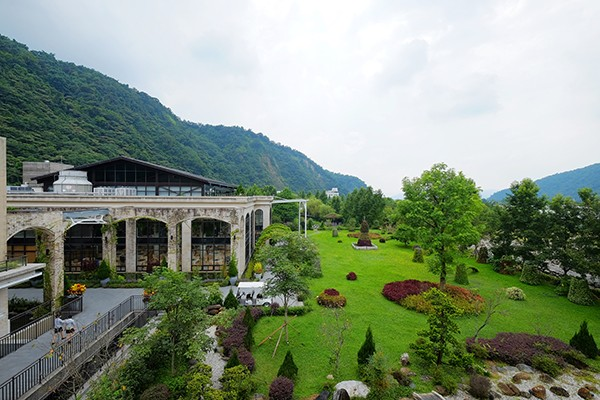環境
•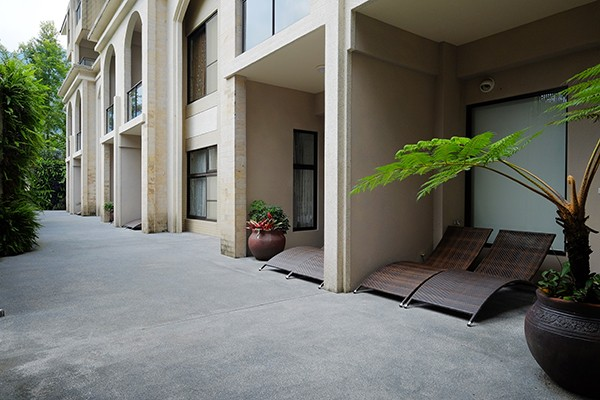環境
•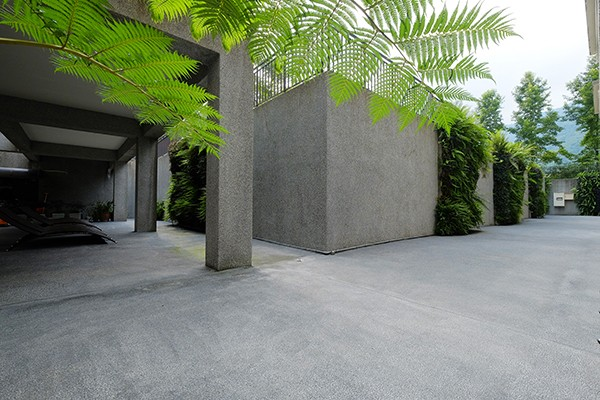環境
•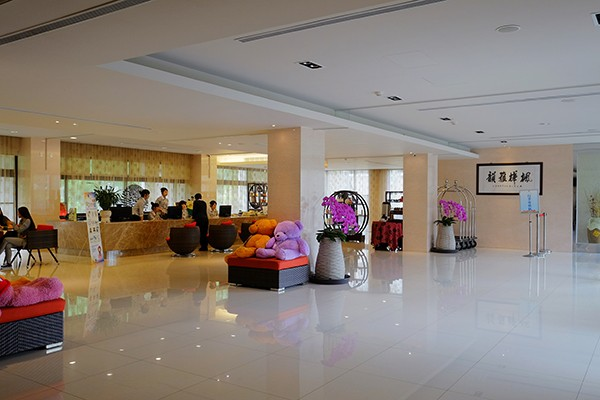環境
•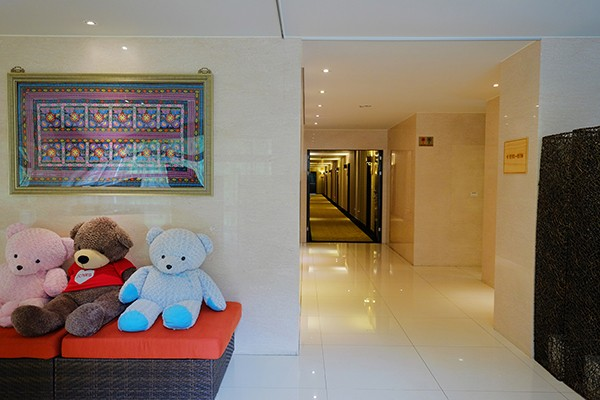環境
•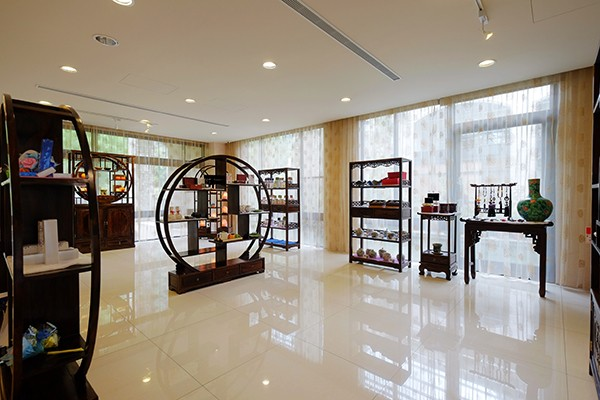環境
•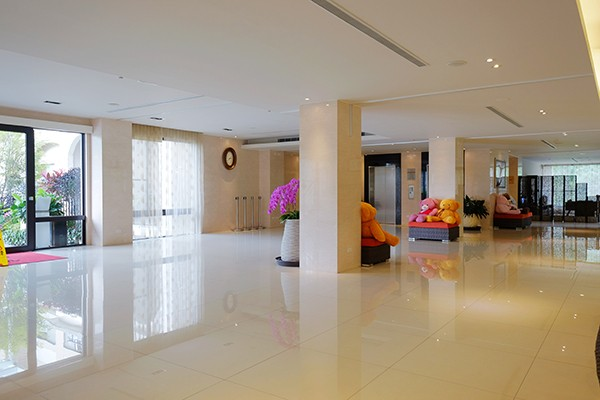環境
•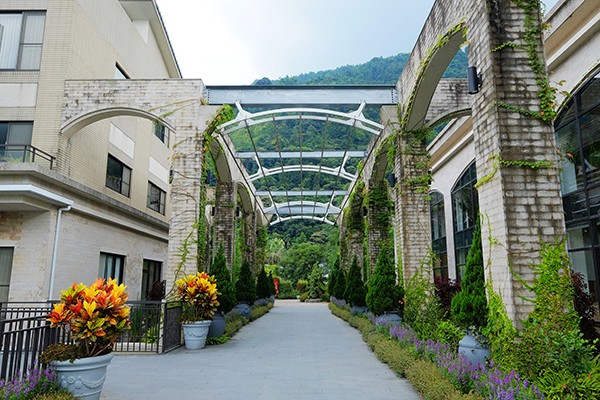環境
•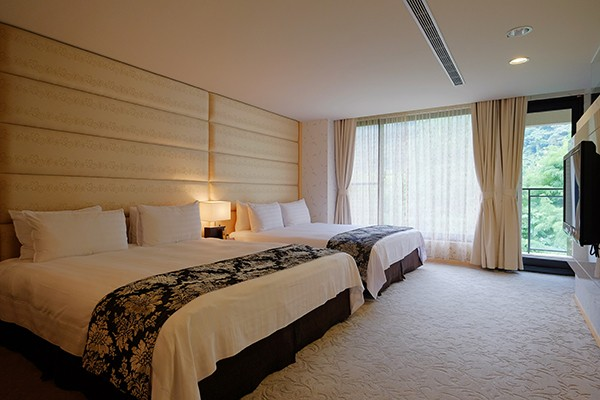環境
•客房
•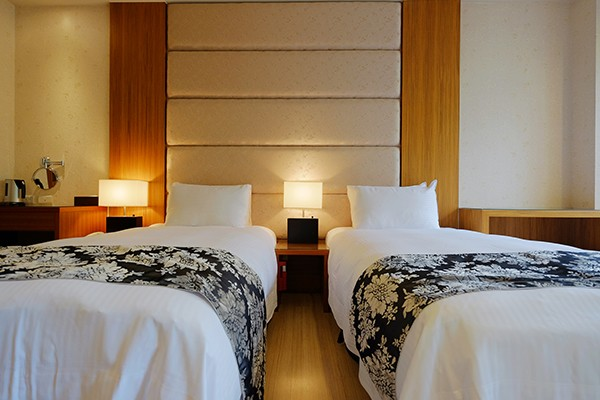客房
•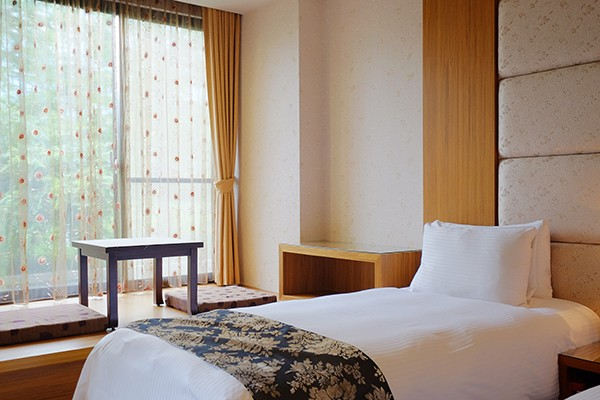客房
•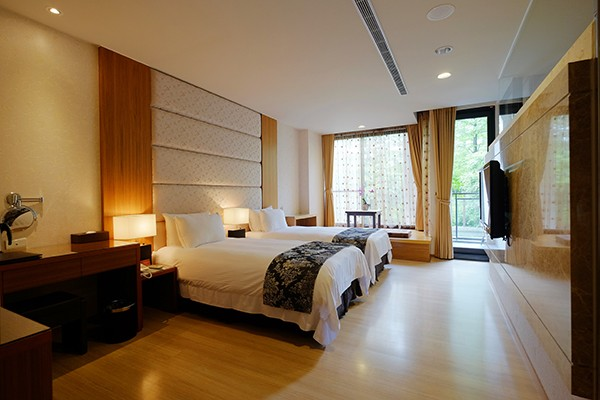客房
•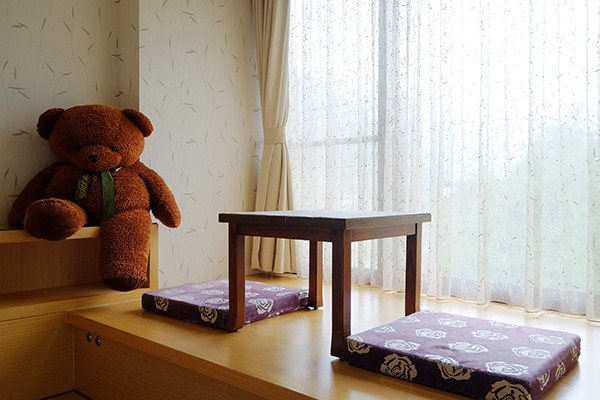客房
•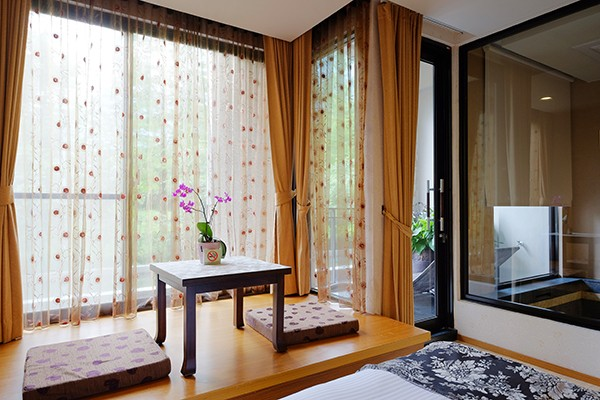客房
•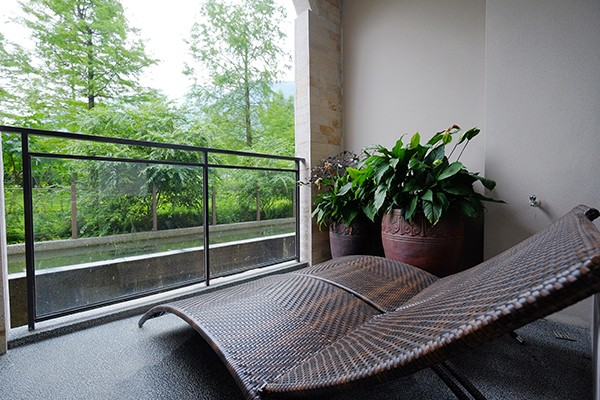客房
•客房
•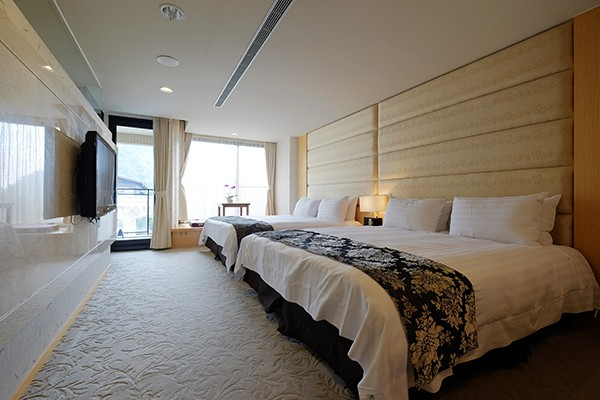客房
•客房
•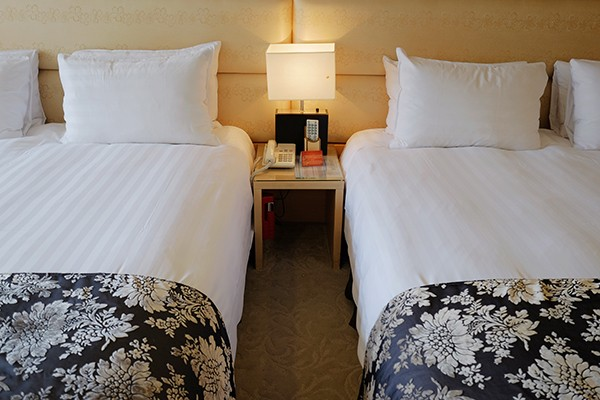客房
•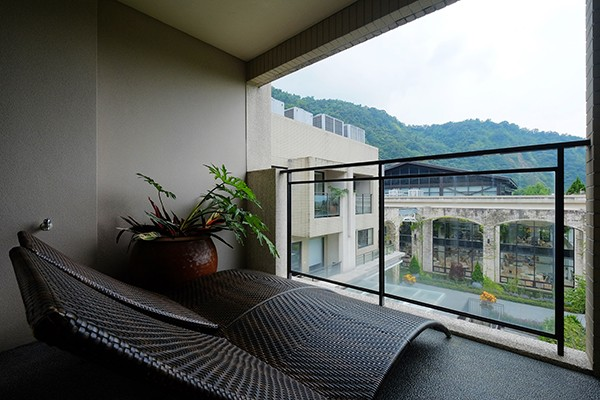客房
•客房
•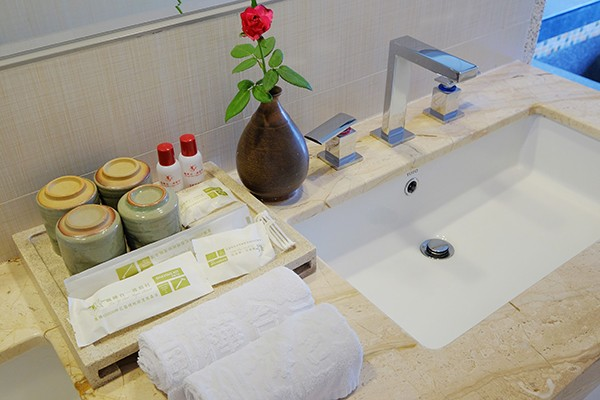客房

#### 飯店設有　 飯店未設 飯店設施

• 24小時客房服務
• 商務中心
• 洗衣服務/乾洗
• Wi-Fi in public areas
• 夜總會
• 禮賓接待服務
• 代客泊車
• 娛樂場
• 自行車出租服務
• 保管服務
• 客房服務
• 行政樓層
• 停車場
• 家庭房
• 酒吧
• 兒童看護服務
• 接送服務
• 電梯
• 可帶寵物
• 旅遊服務
• 飯店/機場接送
• 吸煙房
• 會議設施
• 餐廳
• 咖啡店
• 殘障人士設施
• 髮廊
• 商店
• 池畔吧

• DVD/CD播放器
• 小廚房
• 無線上網(收費)
• 休憩區
• 微波爐
• 燙衣板
• 免費瓶裝水
• 房內保險箱
• 獨立淋浴間
• 公共浴室
• 房內遊戲機
• 獨立用餐區
• 冰箱
• 手提電腦保險箱
• 禁煙房
• 冷氣
• 按摩浴缸
• 私人泳池
• 加長的床(>2m)
• 日報
• 茶與咖啡沖泡設備
• 可提供相連房間
• 書桌
• 衛星頻道/有線電視
• 吹風機
• 洗衣機
• 迷你吧
• 寬頻上網
• 浴室
• 陽台/露台
• 寬頻上網(免費)
• 浴缸
• 電影點播服務
• 寬頻上網(收費)
• 浴袍
• 電視
• 寬頻上網(無線)
• 液晶電視
• 電風扇
• 尊用休息室
• 無線上網(免費)

• spa
• 三溫暖/水療設備
• 網球場
• 健身房/健身設施
• 水上活動
• 花園
• 兒童娛樂室
• 水上運動項目(非機動項目)
• 蒸浴室
• 壁球場
• 溫泉
• 遊戲室/娛樂場
• 室內游泳池
• 游泳池(兒童)
• 高爾夫球場(3km內)
• 室外游泳池
• 漩渦按摩浴池
• 高爾夫球場(飯店)
• 按摩
• 私人海灘

#### 飯店簡介

```座落在台14線上（國道六號底）通往霧社、清境路上最美麗的花卉森林城堡『楓樺台一渡假村』，

73間花泉客房均有大觀景窗，佔約12~20坪空間大小的各式房型，

#### 飯店條款

• 加床政策根據您所選定的客房而有所不同，更多詳情請查看您所選定的客房的政策

#### 相關資訊

退房時間 11:00 AM 2000TWD 5Km 3:00 PM

0
0